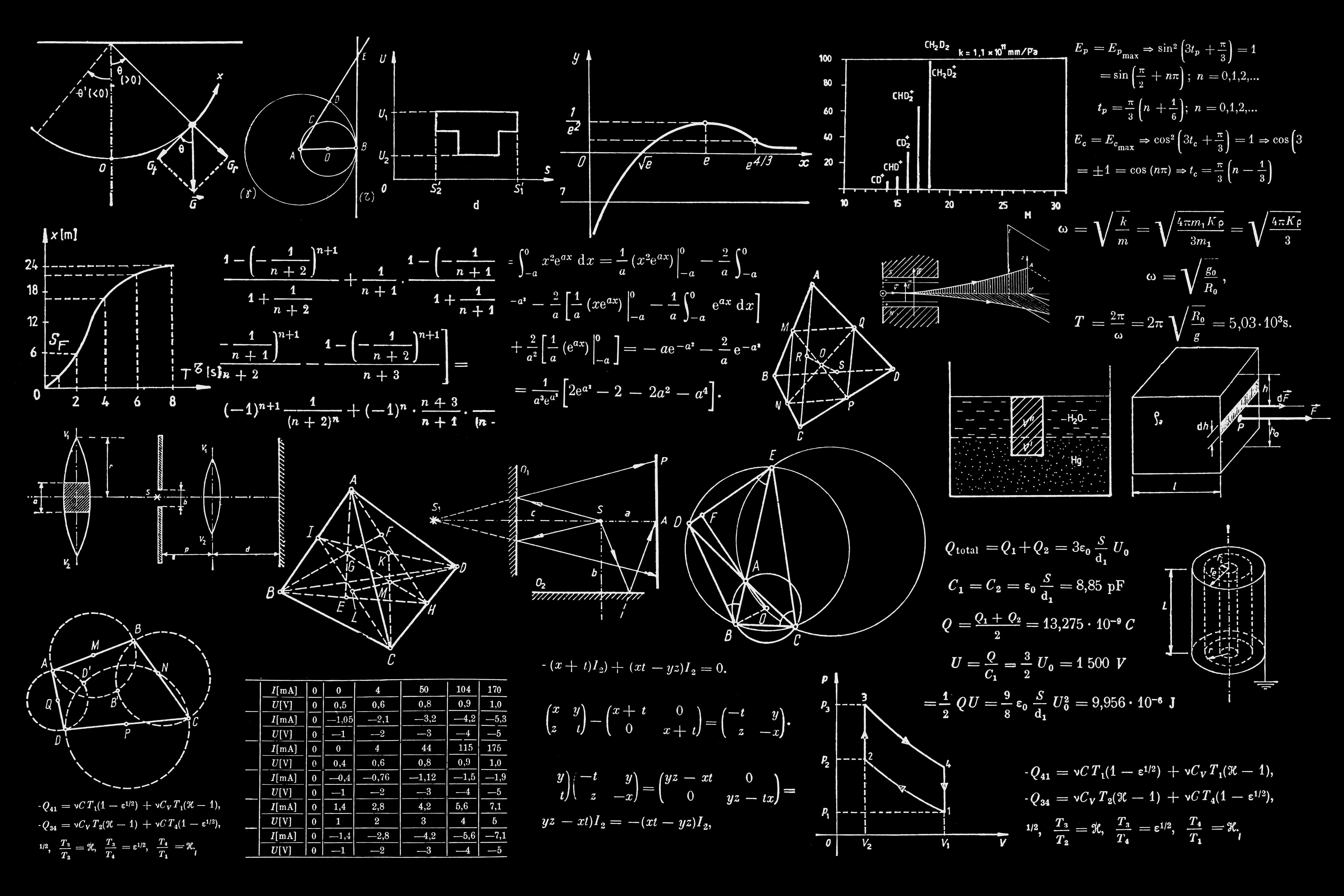Share
Explore#Probability Calculator

If A and B are two events in a sample space S, then the conditional probability of A given B is defined as P(A|B)=P(A∩B)P(B), when P(B)>0.

The calculator uses the input number set to determine the probability of a specific event occurring at a specific location. The output represents the likelihood of that event occurring based on the statistical analysis of the number set

Set Size:
Please Enter Parameters Above To Calculate Probability

Share